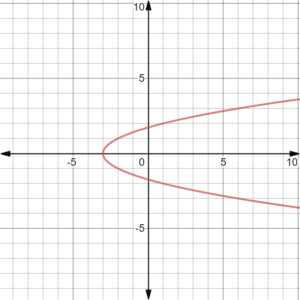As mentioned before, all functions can only have one y-value for each corresponding x-value. Any continuous graph that has more than one y-value for a single x-value is not a function but is a relation. After you finish this lesson, view all of our Algebra 1 lessons and practice problems.

## Example of Formalizing Relations and Functions

### Example 1

Is the following relation shown a function?$(6, 5), (4, 3), (6, 4), (5, 8)$

The answer is no because the$x$ value which is$6$ came out twice.

### Example 2Now, we do the vertical line test.This is not a function since the vertical line touches the graph twice.

## Video-Lesson Transcript

Let’s start by drawing a function machine.

This is a function machine for$f (x)$.

When you say$f$ of$x$, this means that the function is dependent on the value of$x$.

For example:$f (x) = x + 3$

Let’s draw a table which consists of$x$ values and$y$ values or$f (x)$.

So if we put$0$ in the function machine, it will come out$3$.$x = 0$ then$f (x) = 3$

Keep on going and we’ll have$x = 1$ then$f (x) = 4$$x = 2$ then$f (x) = 5$$x = 3$ then$f (x) = 6$$x = 4$ then$f (x) = 7$

The most important thing to remember for functions is for every$x$ value, you will have at most one$y$ value.

Let’s look at this on a graph.

Plot the points on a graph.

We’ll have a straight slash line on the graph.

Let’s look at some more.

Let’s graph the$f(x) = x^2$ and$f(x) = \sqrt{x}$

If we look at these graphs, one$x$ value has one corresponding$y$ value.

Let’s do a vertical line test on these graphs.

Vertical line test tests if a graph is indeed a function.

To do a vertical line test, we have to draw a vertical line at different places on the graph.

If the vertical line intersects the graph at only one point every time, then that is a function.

Even if the vertical line doesn’t touch the graph, it’s okay.

As a rule, we can have at most one$y$ value per$x$ value. So if there is no$y$ value, that’s fine.

But we can not have two$y$ values at the same time.

Let’s draw another graph with a line that has a lot of curves.

You’ll see that at one point on the graph, the vertical line touched the graph multiple times.

This means for one$x$ value there are three$y$ values.

Therefore, this is not a function.Let’s look at a graph of a circle.

If we do the vertical line test here, the vertical line touches the graph twice. So this is not a function.

Now, we’ll have a special graph – an absolute value graph.

If we do the vertical line test on this one, we will see that the vertical line touches the graph once at several places on the graph.

This means this is a function.

Remember, for each$x$ value there is only one$y$ value.

Let’s move on to relations.

Relation may have more than one$y$ value for each$x$ value.

For example:$(2, 1)$,$(3, 5)$,$(4, -2)$,$(0, 1)$,$(2, 3)$

In$(2, 1)$ and$(2, 3)$, you can see that$x$ value$2$ came out twice.

Therefore, this is not a function but it is a relation.

But if let’s say we change$(2, 3)$ into$(6, 3)$ then this will be a function.